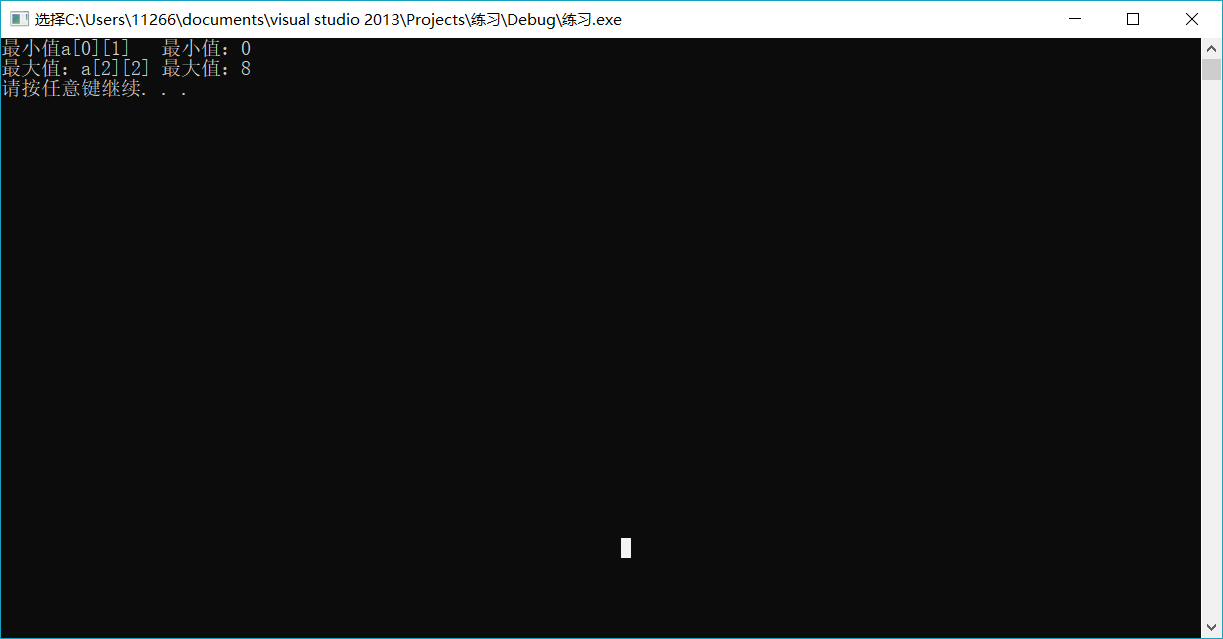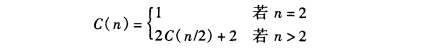• ## java求数组中最大值最小值

万次阅读 多人点赞 2017-07-18 22:22:11
算法描述 默认最大值是第一个元素 默认最小值也是第一个元素 遍历数组元素,比较数组元素的值和默认的最大值,... 另一种方式是记下最大值最小值在数组中的下标，再通过下标输出最大最小值，推荐使用这种方式. 代码...
算法描述

默认最大值是第一个元素

默认最小值也是第一个元素

遍历数组元素,比较数组元素的值和默认的最大值,如果数组元素的值比默认最大值还要大,则用数组元素的值作为默认的最大值,这样遍历一遍之后就可以求出最大值了.求最小值也是同样.

这里有两种方式来求记录最大最小值:

一种是记下最大值和最小值
另一种方式是记下最大值最小值在数组中的下标，再通过下标输出最大最小值，推荐使用这种方式.
代码

具体看下面的代码

public class MaxAndMin {
public static void main(String[] args) {
int[] array={1,3,67,34,23,89,56,34,21};//定义静态数组
System.out.println("----------方法1：-----------");
int max=array;//默认第一个最大
int min=array;//默认第一个最小
for(int i=1;i<array.length;i++)
{
if(array[i]>max)
{
max=array[i];//如果有比max大的数就让max记录下大的数
}
if(array[i]<min)
{
min=array[i];//如果有比min小的数就让min记录下小的数
}

}
System.out.println("max="+max);
System.out.println("min="+min);
System.out.println("---------------方法2：--------------");
int maxlocation=0;//默认第一个最大
int minlocation=0;//默认第一个
for(int i=0;i<array.length;i++)
{
if(array[i]>array[maxlocation])
{
maxlocation=i;//如果有比max大的数就让max记录下大的数
}
if(array[i]<array[minlocation])
{
minlocation=i;//如果有比min小的数就让min记录下小的数
}
}
System.out.println("max="+array[maxlocation]+" 位置："+maxlocation);
System.out.println("max="+array[minlocation]+" 位置："+minlocation);
}
}


展开全文• 思路：先找出最小值，其中*（*（a+i）+j）是二维数组的第i行第j列，然后再找出最大值，办法和最小值一样，找出最大值最小值后，用m和n记录最大值和最小值的位置。 程序比较简单，代码如下： #include<stdio.h&...
 知识点：用指针找出二维数组中的最大值和最小值，并输出其所在的位置。

思路：先找出最小值，其中*（*（a+i）+j）是二维数组的第i行第j列，然后再找出最大值，办法和最小值一样，找出最大值最小值后，用m和n记录最大值和最小值的位置。

程序比较简单，代码如下：

#include<stdio.h>
#include<windows.h>
void hanshu(int(*a))
{
//最小
int i, j, m, n, max, min;
min = *(*(a + 0) + 0);
for (i = 0; i < 3; i++)
{
for (j = 0; j < 3; j++)
{
if (min > *(*(a + i) + j))
{
min = *(*(a + i) + j);
m = i; n = j;
}
}
}

printf("最小值a[%d][%d]\t最小值：%d\n", m, n, min);
//最大
max = *(*(a + 0) + 0);
for (i = 0; i < 3; i++)
{
for (j = 0; j < 3; j++)
{
if (max < *(*(a + i) + j))
{
max = *(*(a + i) + j);
m = i; n = j;
}
}
}
printf("最大值：a[%d][%d]\t最大值：%d\n",m,n,max);
}
void main()
{
int a = { { 2, 0, 1 }, { 6, 3, 4 }, { 5, 7, 8 } };
hanshu(a);
system("pause");
}


根据定义的二维数组输出的结果:展开全文• C++ PTA 求最大值最小值 用指针作函数参数，编程序求一维数组中的最大和最小的元素值。 函数接口定义： void maxmin(int arr[ ],int *pt1,int *pt2,int n); 其中 arr、 pt1、pt2、n都是用户传入的参数，n为元素个数...
C++ PTA 求最大值最小值
用指针作函数参数，编程序求一维数组中的最大和最小的元素值。
函数接口定义：
void maxmin(int arr[ ],int *pt1,int *pt2,int n);

其中 arr、 pt1、pt2、n都是用户传入的参数，n为元素个数。函数求指针arr所指向的一维数组中的最大和最小的元素值，并将最大值和最小值存入指针pt1和pt2所指的存储单元中。
裁判测试程序样例：
#include<stdio.h>
#define N 10
void maxmin(int arr[ ],int *pt1,int *pt2,int n);
int main( )
{ int array[N]={10,7,19,29,4,0,7,35,-16,21},*p1,*p2,a,b;
p1=&a;p2=&b;
maxmin(array,p1,p2,N);
printf("max=%d,min=%d",a,b);
return 0;
}

/* 请在这里填写答案 */

输出样例：
max=35,min=-16

答案：
void maxmin(int arr[], int *pt1, int *pt2, int n) {
*pt1 = *pt2 = arr;
for(int i = 1; i < n; i++) {
if(arr[i] > *pt1) *pt1 = arr[i];
if(arr[i] < *pt2) *pt2 = arr[i];
}
}



展开全文• 1.问题描述：输入一组数据，找出其中的最大值最小值 2.分治思想： 找数组a范围l~R的最值=> ①范围一分为2 mid=(l+r)/2 ②最大值=max(l~mid最大值，mid+1~r最大值)； ③最小值=min(l~mid最小值...
1.问题描述：输入一组数据，找出其中的最大值最小值

2.分治思想：

找数组a范围l~R的最值：

①范围一分为2 mid=(l+r)/2

②最大值=max(l~mid最大值，mid+1~r最大值)；

③最小值=min(l~mid最小值，mid+1~r最小值)；

边界：

只有一个元素，最大值=最小值=a[l]

3.代码实现:

#include <iostream>
#include <fstream>
#include <windows.h>
using namespace std;
void search(int *a, int l,int r, int &maxi,int &mini){
if(l==r){
maxi=mini=a[l];return;
}
int mid=(l+r)/2;
int max1,max2,min1,min2;
search(a,l,mid,max1,min1);
search(a,mid+1,r,max2,min2);
maxi=max(max1,max2);
mini=min(min1,min2);
}
int main(){
int n,i,maxi,mini;
LARGE_INTEGER nFreq,nBegin,nEnd;
double time;

ifstream in("input.txt");
ofstream out("output.txt");
in>>n;
int a[n];
for(i=0;i<n;i++)
in>>a[i];

QueryPerformanceFrequency(&nFreq);
QueryPerformanceCounter(&nBegin);
search(a,0,n-1,maxi,mini);
QueryPerformanceCounter(&nEnd);
time=(double)(nEnd.QuadPart-nBegin.QuadPart)/(double)nFreq.QuadPart;

out<<"maxi:"<<maxi<<" mini:"<<mini<<"\n search time:"<<time<<"s\n";
in.close();
out.close();
return 0;
}

②功能函数：生成规模为n的随机数

#include <time.h>
#include <stdlib.h>
#include <fstream>
#include <iostream>
using namespace std;
int main(){
int n;
cin>>n;
ofstream out("input.txt");
out<<n<<'\n';
srand((unsigned)time(NULL));
for(int i=0;i<n;i++){
out<<rand()<<' ';
if((i+1)%10==0) out<<'\n';
}
out.close();
return 0;
} 

4.复杂度分析：

①主函数：

时间复杂度分析：

search函数：

递推关系：①利用主定理：

发现和遍历一样是O（n)的

②迭代

③与遍历比较：

5.测试数据及结果分析：

运行时间与规模
规模n
10
100
1000
10000
100 000
500 000
耗时/s
1.48e-005
1.7e-005
6.01e-005
6.542e-4
6.7138e-3
4.0174e-2


展开全文算法 分治算法
• Java语言用函数实现返回数组中最大值最小值和平均值，请给我代码 Java语言用函数实现返回数组中最大值最小值和平均值，请给我代码 Java语言用函数实现返回数组中最大值最小值和平均值，请给我代码开发语言
• 1 # -*- coding: utf-8 -*- 2 """ 3 Created on Fri Sep 7 16:28:20 2018 4 ... 7 # 最大值最小值归一化:(X-Xmin)/(Xmax-Xmin) 8 import numpy as np 9 import matplotlib.pyplot as ...
• 最大值最小值位置 从键盘任意输入10个整数，计算并输出最大值和最小值及其它们在数组中的下标位置。 程序运行结果示例1： Input 10 numbers:1 2 3 4 5 6 7 8 9 10↙ max=10,pos=9 min=1,pos=0 程序运行结果...c语言
• 一、问题描述 学校的学生的是一个非常大的生成数据的集体，比如每次考试的成绩。现有一个班级的学生一个月的考试成绩...在reduce阶段进行最大值最小值平均值得求值。 三、代码实现 1、Map阶段 package com.stude...MapReduce
• 定理1：有界性与最大值最小值定理 在闭区间上连续的函数&&该区间上有界，一定能取得最大值和最小值。 关键字：比区间、连续、有界。 定理2：零点定理 如果x0使f(x0)=0，那么x0称为函数f(x)的零点。 设函数f...
• print(a.index(max(a))) # 返回第一个最大值的位置 print(np.max(b)) # 返回最大值 print(np.min(b)) # 返回最小值 print(np.argmax(b)) # 返回第一个最小值的位置 print(np.argmin(b)) # 返回第一个最大python
• 输入一组5个float类型的数据，要求输出这组数据，并且输出这组数据的平均值 最大值 最小值。 格式： 输入第 1 数据: 45 输入第 2 数据: 55 输入第 3 数据: 60 输入第 4 数据: 65 输入第 5 数据: 70 这组数据为:45.0 ...
• 同时查找最大值最小值优化算法 本算法并不是直接拿数组中的元素来和最大值和最小值比较的，而是先比较数组中两个数组，然后那其中小的和最小值对比，其中大的和最大值对比，那么算法就可以由原来需要对比2n次，降到...算法
• 最大值最小值问题 两个数的最值问题 两个数的比较问题很简单，主要有以下方法： 直接使用if语句判断。 >>int a = 1,b = 2,max,min; >>if(a>b) {max = a;min = b;} >>else {max = b;...c++
• Hadoop 求最大值 最小值   1、源代码 package com.dtspark.hadoop.hellomapreduce; import java.io.IOException; import java.util.Iterator; import java.util.StringTokenizer;   import org.apache....
• C++数组、vector求最大值最小值和下标 可以用max_element（）及min_element（）函数，二者返回的都是迭代器或指针。 头文件： #include<algorithm> 一.求数组的最大值或最小值 1、vector容器 例 ...
• Oracle中取某几个数的最大值最小值 一直有误区认为Oracle或者说关系型数据库中没有取某些数据中最大值最小值的函数，其实是存在的 分别为： greatest( expr1, expr2, ... expr_n ) least( expr1, expr2, ... expr_n ...
• layui.laydate 动态设置最大值最小值 一般设置最大、最小值是这么设置的，直接给max赋值一个字符串 laydate.render({ elem: '#beginTime', type: 'datetime', btns: ['confirm','clear'], format: 'yyyy-MM-dd ...
• c++数组或vector容器求最大值最小值 可以用max_element（）及min_element（）函数，二者返回的都是迭代器或指针。 头文件：#include< algorithm > #include < vector > 1.求数组的最大值或最小值 例 a[]...c++
• 方法1：遍历两次求出最大值最小值 ，时间复杂度N*2 方法2：相邻两个数分为一组比较，大的放在偶数位，小的放到奇数位，然后在偶数位上找到最大值，在奇数位上找到最小值N*1.5 方法3：定义两个变量Max，Min值，相邻...
• public static void main(String[] args) { //动态录入5个数据，求平均值 最大值 最小值 Scanner sc =new Scanner(System.in); int[] arr =new int; int total =0; for (int i = 0; i <...
• 7-2 最大值最小值 (10分) 键盘输入三个整数，要求输出其最大值和最小值。 输入格式: 键盘输入只有一行，即三个整数，每个整数之间一个空格。 输出格式: 用一行输出最大值和最小值，之间用一个空格隔开。 输入样例: ...c语言
• /**求一个数组的最大值最小值及其下标 思路:假定一个数为最大值,如果有个数比假定的最大值还大,那么该数就为最大值. 最小值同理. 使用for循环. */ public class MaxMin{ public static void main(String[] args){...最小元素下标
• Description 编制函数，其功能是在float类型一维数组中查找最大值、最小值，并将它们返回到调用程序。.../*找出float数组中的最大值最小值并返回主函数*/ #include<stdio.h> float max(float *n,int算法
• python求列表中数字的最大值最小值及下标 a = [2,3,4,5,6,7] max_num = a min_num = a max_index = 0 min_index = 0 for i in range(len(a)): if a[i]>max_num: max_num = a[i] max_index = i else...
• js移出数组中的最大值最小值 Math.max(...arr) Math.min(...arr) arr,splice()
• 获取集合中最大值最小值 一、 普通集合 1、 查询集合中字符串的个数最多和最少 List<String> strs = new ArrayList<String>(); strs.add("a"); strs.add("ab"); strs.add("qwrrrrrrr"); ...java
• InputNumber 数字输入框限制输入不为空，最大值最小值 Ant-Design-Vue 数字输入框组件InputNumber 限制用户只能输入数字，且不能为空，限制输入最大值最小值 关键字：parser，指定从 formatter 里转换回数字的方式，...数字输入框 parser
• MySQL 获取多个字段最大值最小值   greatest(字段1，字段2，字段3，..，字段n) 取最大值 least(字段1，字段2，字段3，...，字段n) 取最小值
• js获取数组最大值最小值max,min 点击查看文章内容 数组排序，获取第一个和最后一个为最小值最大值;此方法通用 var arr=[2,3,5,6]; var sortArr=arr.sort(function(a,b){return a-b;}); var min=sortArr; var ...js数组排序...